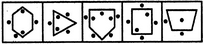# Non Verbal Reasoning - Classification - Discussion

### Discussion :: Classification - Section 1 (Q.No.37)

In each problem, out of the five figures marked (1), (2), (3), (4) and (5), four are similar in a certain manner. However, one figure is not like the other four. Choose the figure which is different from the rest.

37.

Choose the figure which is different from the rest.(1)     (2)     (3)     (4)     (5)

 [A]. 1 [B]. 2 [C]. 3 [D]. 4 [E]. 5

Explanation:

In all other figures, the number of dots outside the main figure is one more than the number of dots inside the main figure.

 Kanchan said: (Jun 27, 2011) But in figure 4 no of dots outside are 2 more than the dots inside the figure.

 Dev said: (Aug 22, 2011) You r right Kanchan. So, the ans is D because it ask about different from the rest.

 Priya said: (Aug 30, 2013) Answer is A because in all other option we fold the paper and they are mirror image of each other but that is not possible in case of A.

 Tod said: (Apr 11, 2014) D could have also been the correct answer because the rectangle has no slanted lines unlike the rest of the shapes.

 Animesh Chincholikar said: (Oct 6, 2016) Here, the answer is inappropriate. It must be 1. As the no of dots are 1 less than the sides of the figure. So, Answer is 1.

 Anshu Krishnadutta Sharma said: (Dec 12, 2016) Answer is d because in 1st total no dots is 5 in second total no of dots is 5. In 3rd total no of dots is 7 and in 5th total no of dots are 3 all dots are odd in no's but in fourth no of dots is 6 that is even so d is answer. So except d option other option consists odd no of dots i.e. 5, 5, 7, 3 and d consists 6. i.e even no so d is the answer.

 Vidhi said: (Feb 25, 2017) Then why it is not option 3?

 Imran said: (Dec 17, 2017) Answer 3 is correct because each figures have no side is exactly divisible by no of dots outside.

 Sweetybhadoria said: (Aug 8, 2018) Option E is also correct because the dot is at the centre and no other figure has a dot at the centre.

 Srija said: (Dec 25, 2018) Total no of dots are odd except fig D. So, the answer should be option D.

 Abhishek said: (Jun 8, 2019) In my opinion, in picture 4 there is 4 dot means even numbers. Other pictures dots are odd numbers.

 Charmaine said: (Nov 14, 2020) Its D because the 6 dots and is an even number the rest of the dots are odd numbers.